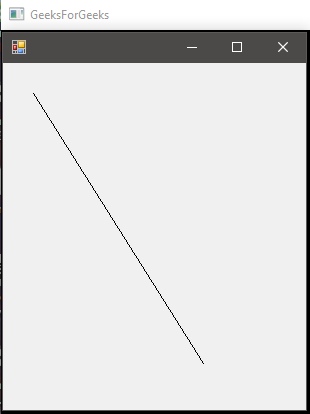# C# | Graphics.DrawLine() Method | Set – 2

Graphics.DrawLine() Method is used to draw a line connecting the two points specified by the coordinate pairs. There are 4 methods in the overload list of this method as follows:

• DrawLine(Pen, PointF, PointF) Method
• DrawLine(Pen, Int32, Int32, Int32, Int32) Method
• DrawLine(Pen, Single, Single, Single, Single) Method
• DrawLine(Pen, Point, Point) Method

First, two methods are already discussed in Set – 1. Here, we will discuss the last two methods.

#### DrawLine(Pen, Single, Single, Single, Single) Method

This method is used to draw line form a specified set of coordinates given in form x1, y1, x2, y2 all discrete.

Syntax:

public void DrawLine (System.Drawing.Pen pen, float x1, float y1, float x2, float y2);

Parameters:

• pen: Pen determines the color, width, and style of the line.
• x1: The abscissa of first point.
• y1: The ordinate of first point.
• x2: The abscissa of second point.
• y2: The ordinate of second point.

Exception: This method will give ArgumentNullException if the pen is null.

Example:

 `// C# program to illustrate the use of  ` `using` `System; ` `using` `System.Drawing; ` `using` `System.Drawing.Printing; ` `using` `System.Windows.Forms; ` ` `  `namespace` `GFG { ` ` `  `class` `PrintableForm : Form { ` ` `  `    ``// Main Method ` `    ``public` `static` `void` `Main() ` `    ``{ ` `        ``Application.Run(``new` `PrintableForm()); ` `    ``} ` ` `  `    ``public` `PrintableForm() ` `    ``{ ` `        ``ResizeRedraw = ``true``; ` `    ``} ` ` `  `    ``protected` `override` `void` `OnPaint(PaintEventArgs pea) ` `    ``{ ` `        ``// Defines the Pen ` `        ``Pen pen = ``new` `Pen(ForeColor); ` ` `  `        ``// x1 = 30 ` `        ``// y1 = 30 ` `        ``// x2 = 200 ` `        ``// y2 = 300 ` `         `  `        ``// using the Method ` `        ``pea.Graphics.DrawLine(pen, 30.0F, 30.0F, 200.0f, 300.0f); ` `    ``} ` `} ` `} `

Output:#### DrawLine(Pen, Point, Point)

This method is used to draw a line from a specified set of points to a specified set of points. It needs a Point variable which is set of (x, y) points.

Syntax:

public void DrawLine (System.Drawing.Pen pen, System.Drawing.PointF pt1, System.Drawing.PointF pt2);

Parameters:

• pen: Pen determines the color, width, and style of the line.
• pt1: Defines the (x, y) coordinates as a Point variable of the initial point.
• pt2: Defines the (x, y) coordinates as a Point variable of the final point.

Exception: This method will give ArgumentNullException if the pen is null.

Example:

 `// C# program to demonstrate the use of ` `// DrawLine(Pen, Point, Point) Method ` `using` `System; ` `using` `System.Drawing; ` `using` `System.Drawing.Printing; ` `using` `System.Windows.Forms; ` ` `  `namespace` `GFG { ` ` `  `class` `PrintableForm : Form { ` ` `  `    ``// Main Method ` `    ``public` `static` `void` `Main() ` `    ``{ ` `        ``Application.Run(``new` `PrintableForm()); ` `    ``} ` ` `  `    ``public` `PrintableForm() ` `    ``{ ` `        ``ResizeRedraw = ``true``; ` `    ``} ` ` `  `    ``protected` `override` `void` `OnPaint(PaintEventArgs pea) ` `    ``{ ` `        ``// Defines pen ` `        ``Pen pen = ``new` `Pen(ForeColor); ` ` `  `        ``// Defines the both points to connect ` `        ``// pt1 is (30, 30) which represents (x1, y1) ` `        ``Point pt1 = ``new` `Point(30, 30); ` ` `  `        ``// pt1 is (200, 300) which represents (x2, y2) ` `        ``Point pt2 = ``new` `Point(200, 300); ` ` `  `        ``// Draws the line ` `        ``pea.Graphics.DrawLine(pen, pt1, pt2); ` `    ``} ` `} ` `} `

Output:My Personal Notes arrow_drop_upCheck out this Author's contributed articles.

If you like GeeksforGeeks and would like to contribute, you can also write an article using contribute.geeksforgeeks.org or mail your article to contribute@geeksforgeeks.org. See your article appearing on the GeeksforGeeks main page and help other Geeks.

Please Improve this article if you find anything incorrect by clicking on the "Improve Article" button below.

Article Tags :

Be the First to upvote.

Please write to us at contribute@geeksforgeeks.org to report any issue with the above content.